# 铝合金电磁铆接接头疲劳性能研究Research on Fatigue Properties of Electromagnetic Riveted Joints for Aluminum Alloy Structures

DOI: 10.12677/MS.2019.97082, PDF, HTML, XML, 下载: 508  浏览: 770  科研立项经费支持

Abstract: In this paper, the shear and fatigue properties of electromagnetic riveting joints were studied for 2A10 aluminum rivets and ZL114A cast aluminum sheets commonly used in aerospace. The shear test results showed that the maximum shear load of electromagnetic riveted joints with aluminum alloy rivets of 4 mm diameter was about 3.5 kN. The failure position was at the junction of two sheets on rivet rod. The fatigue test results showed that the failure mode of the specimens was the fracture on the rivet rod, which was similar to the shear failure mode. In the failure process, fatigue crack initiated from the edge of rivet rod, and brittle fracture occurred when fatigue crack extended to one third of rivet rod. In addition, the fatigue data were analyzed statistically, and the fatigue life under different reliability levels was obtained. It could provide design reference for the application of electromagnetic riveted joints.

1. 引言

2. 试验材料及方法

2.1. 试件准备Table 1. Composition of ZL114A cast aluminum sheet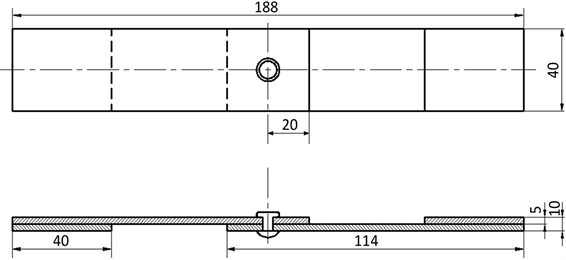Figure 1. Schematic of dimensions for the riveted specimen

2.2. 电磁铆接试验设备

2.3. 测试方法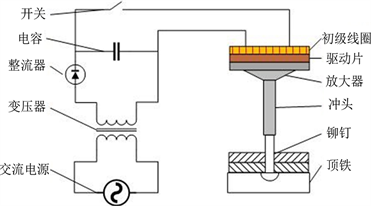(a) 电磁铆接原理示意图(b) 手持式电磁铆枪

Figure 2. Principle and equipment of electromagnetic riveting

3. 试验结果及分析

3.1. 剪切性能分析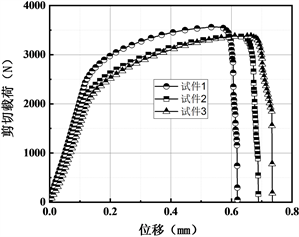(a) 剪切载荷–位移曲线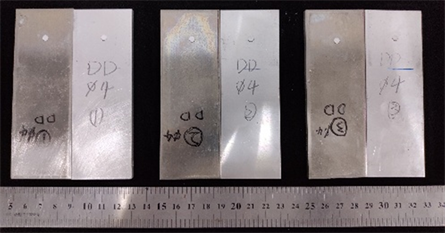(b) 剪切失效试件

Figure 3. Results of shear tests

3.2. 疲劳寿命分析

$f\left(t\right)=\frac{\beta }{\eta }{\left(\frac{t}{\eta }\right)}^{\beta -1}\mathrm{exp}\left[-{\left(\frac{t}{\eta }\right)}^{\beta }\right]$ (1)

$F\left(t\right)=1-\mathrm{exp}\left[-{\left(\frac{t}{\eta }\right)}^{\beta }\right]$ (2)

β和η分别代表形状和尺度参数；t表示疲劳寿命。可靠度函数定义为：

$R\left(t\right)=\mathrm{exp}\left[-{\left(\frac{t}{\eta }\right)}^{\beta }\right]$ (3)

$\mathrm{ln}\mathrm{ln}\frac{1}{R\left(t\right)}=\beta \mathrm{ln}\left(t\right)-\beta \mathrm{ln}\eta$ (4)

$\stackrel{˙}{R}\left({t}_{i}\right)=1-\frac{i-0.3}{n+0.4}\text{\hspace{0.17em}}\text{\hspace{0.17em}}\text{\hspace{0.17em}}\left(i=1,2,\cdots ,n\right)$ (5)Table 2. Fatigue test results of electromagnetic riveted joints

Continued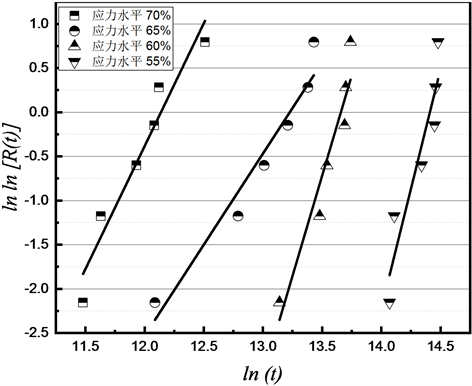Figure 4. Weibull distribution probability diagramTable 3. Weibull distribution parameter results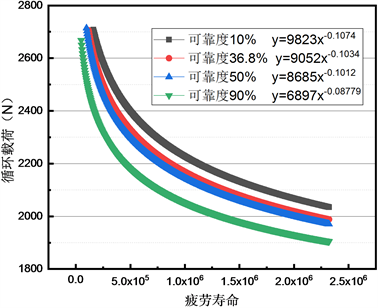Figure 5. S-N curves at different reliability levels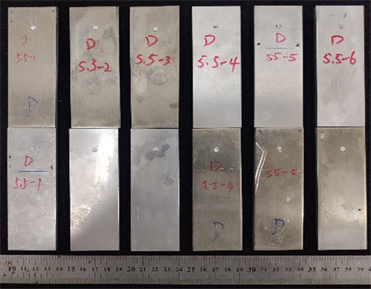(a) 应力水平55%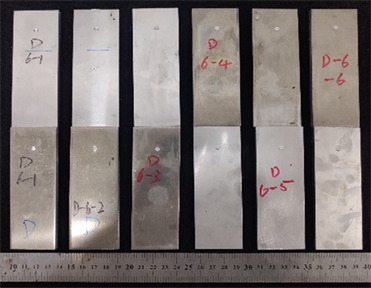(b) 应力水平60%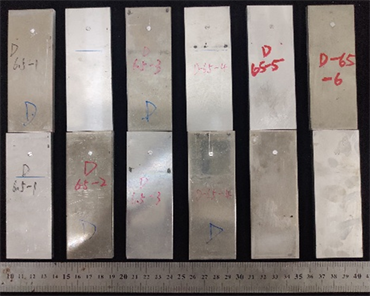(c) 应力水平65%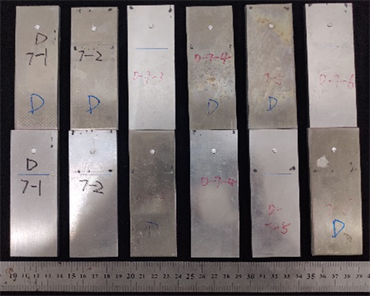(d) 应力水平70%

Figure 6. Fatigue failure modes of electromagnetic riveted joints

3.3. 断口分析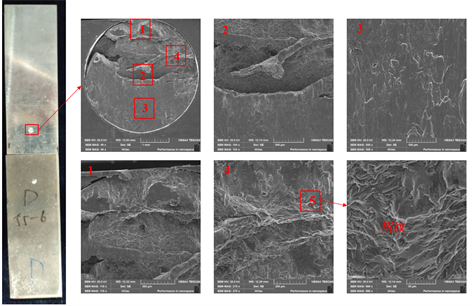Figure 7. Fatigue fracture of electromagnetic riveted joint

4. 结论

1) 直径4 mm铝合金铆钉电磁铆接接头的最大剪切载荷大概3.5 kN。失效位置位于铆钉钉杆两板的交界处。这是因为剪切过程中，其他位置由于有孔壁的约束会抵消部分剪切应力，而两板交界处承受最大的剪切应力。

2) 对疲劳数据进行了统计学分析，得到不同可靠度水平下的疲劳寿命，可对电磁铆接接头的疲劳寿命进行评估，可靠度越高，疲劳循环周期越低。因此，该结果可对不同安全系数下接头的疲劳寿命进行评估，为电磁铆接接头的应用提供设计参考。

3) 在不同应力水平下，试件的失效模式一致，均为铆钉钉杆发生断裂，与剪切测试的失效模式相似。失效过程中，疲劳裂纹由钉杆边缘萌生，扩展至钉杆三分之一处即发生脆性断裂。

  薛红前. 飞机装配工艺学[M]. 西安: 西北工业大学出版社, 2015: 3-4.  曹增强. 应对我国大飞机研制的装配连接技术[J]. 航空制造技术, 2009(2): 88-91.  雷昌毅. 无头铆钉干涉连接工艺研究及铆模结构优化[D]: [博士学位论文]. 杭州: 浙江大学, 2018.  罗通. 电磁铆接干涉量理论分析及工艺试验研究[D]: [硕士学位论文]. 长沙: 湖南大学, 2018.  薛俊, 杜兆才, 秦玉波. 铝合金铆钉电脉冲铆接工艺试验研究[J]. 装备制造技术, 2019(1): 1-5+17.  陈如明, 范治松, 黄伍平, 邓将华. 锪窝及凹模对无头铆钉电磁铆接变形的影响[J]. 塑性工程学报, 2017, 24(5): 25-31.  Zieve, P.B., Rudberg, T., Vogeli, et al. (2004) A Two Tower Riveting Machine with a True Z Axis. SAE Technical Paper Series. https://doi.org/10.4271/2004-01-2807  Hartmann, J. and Macias, E. (1998) ASAT4-Enhanced Flexibility for the C-17. Aircraft Structure. https://doi.org/10.4271/982126  Hiratsuka, N., Osawa, T., Assadi, M., et al. (2007) One Piece Barrel Fas-tening. SAE Technical Paper Series. https://doi.org/10.4271/2007-01-3913  Repetto, E.A., Radovitzky, R., Ortiz, M., Lundquist, R.C. and Sandstrom, D.R. (1999) A Finite Element Study of Electromagnetic Riveting. Journal of Manufacturing Science and Engineering, 121, 61-68. https://doi.org/10.1115/1.2830576  Reinhal, P.G., Ghassaei, S. and Choo, V. (1988) An Analysis of Rivet Die Design in Electromagnetic Riveting. Journal of Vibration & Acoustics, 110, 65-69. https://doi.org/10.1115/1.3269482  Cao, Z. and Cardew-Hall, M. (2006) Interfer-ence-Fit Riveting Technique in Fiber Composite Laminates. Aerospace Science and Technology, 10, 327-330. https://doi.org/10.1016/j.ast.2005.11.003  冯东格, 曹增强. 电磁铆接和锤铆铆接质量对比分析[J]. 锻压技术, 2012, 37(3): 123-126.  Li, G., Jiang, H., Zhang, X., et al. (2017) Mechanical Properties and Fatigue Behavior of Electromagnetic Riveted Lap Joints Influenced by Shear Loading. Journal of Manufacturing Processes, 26, 226-239. https://doi.org/10.1016/j.jmapro.2017.02.022  邓将华, 熊燕, 刘大海, 等. 电磁铆接放电电压对TB3铆钉变形的影响[J]. 塑性工程学报, 2014(2): 124-128.  Jiang, H., Luo, T., Li, G., et al. (2017) Fatigue Life Assess-ment of Electromagnetic Riveted Carbon Fiber Reinforce Plastic/Aluminum Alloy Lap Joints Using Weibull Distribution. International Journal of Fatigue, 105, 180-189. https://doi.org/10.1016/j.ijfatigue.2017.08.026